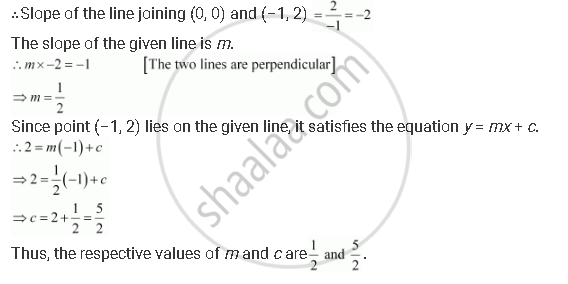CBSE (Arts) Class 11CBSE
Share

# The Perpendicular from the Origin to the Line Y = Mx + C Meets It at the Point (–1, 2). Find the Values of M and C. - CBSE (Arts) Class 11 - Mathematics

ConceptGeneral Equation of a Line

#### Question

The perpendicular from the origin to the line y = mx + c meets it at the point (1, 2). Find the values of and c.

#### Solution

The given equation of line is y = mx + c.

It is given that the perpendicular from the origin meets the given line at (–1, 2).

Therefore, the line joining the points (0, 0) and (–1, 2) is perpendicular to the given line.Is there an error in this question or solution?

#### Video TutorialsVIEW ALL 

Solution The Perpendicular from the Origin to the Line Y = Mx + C Meets It at the Point (–1, 2). Find the Values of M and C. Concept: General Equation of a Line.
S